0% Complete# MINDS ON

Our modern world would not be the same without electricity and magnetism. Electricity is used to light, and in some cases, heat our homes, and in combination with magnetism drives all sorts of technology from electric motors to telephones to trains and automobiles.

#Reflection

Create a reflection to answer the following questions:

1. Take a moment to review your daily routine, from the moment you awaken until you fall asleep at night. List the different pieces of technology that you interact with in a typical day that use electrical and/or magnetic principles to operate.
2. Imagine your day without any of those devices. What changes would this make to your daily routine?# ACTION

The particle theory of matter tells us that all matter is made up of tiny particles called molecules. A three year old might ask their mother what molecules are made of. She could explain that molecules are made up of smaller particles known as atoms. The same three year old might then ask their father what atoms are made of. He could explain that atoms are made up of even smaller particles known as electrons, neutrons, and protons. Electrons and protons carry an electric charge – electrons carry a negative charge and protons carry a positive charge.  Neutrons, as their name suggests, are neutral and do not carry an electric charge. That curious three year old might then ask what electrons, protons, and neutrons are made of, at which point their father would probably tell them to go and ask their mother.

Three subatomic building block particles (electrons, neutrons, and protons) make up all of the matter that you see around you. Inside an atom, protons and neutrons are the most massive particles, and are packed tightly into a small volume at the centre of the atom known as the nucleus. Electrons have a relatively small mass – it would take about 2000 electrons to equal the mass of one neutron or proton. In the Rutherford-Bohr model of the atom, electrons move around the nucleus in circular orbits, in much the same way as the planets orbit the Sun in our solar system. The real physics underlying atoms is more complex, but the Rutherford-Bohr model is simpler and will adequately explain the phenomena we will be exploring.

# Law of Electric Charges

The law of electric charges states that unlike charges are attracted to each other and like charges are repelled from each other. In other words, opposites attract. For the three subatomic building block particles, this means that electrons and protons are attracted to each other, while neutrons are not subjected to any electric forces. If you remember the Rutherford-Bohr model of the atom being much like the solar system, the main difference is that the force holding the planets in their orbits is gravity while the force holding the electrons in their orbits is the force between the positively charged protons in the nucleus and the negatively charged electrons orbiting it.# The Strong Nuclear Force

If like charges repel each other, then why don’t all those positively charged protons in the nucleus fly apart?

There is another force that is more powerful than the repulsive forces acting to push the protons apart from one another. This force is the strong nuclear force, and it acts over very small distances to hold the positively charged protons together in the nucleus. The protons in the nucleus do experience a small repulsive force between each other due to their like charges, but, they also experience the strong(er) nuclear force holding them together.

The strong nuclear force exists between protons and neutrons in the nucleus. In this sense, you can think of the purpose of neutrons being to “dilute” all of that positive charge, so that there is less repulsive force between the protons, and amplify the strong nuclear force to hold the nucleus together.

# Electron Flow and Conventional Current

Before scientists knew too much about the structure of the atom, they had already discovered a lot about electricity. They theorized that a current of positive charges flowed through conductors such as wires in an electric circuit. This is what is known as “conventional current,” flowing for example from the positive terminal of a battery, through a circuit to the negative terminal of a battery.

Some time after the convention was established that current was the flow of positive charges from positive to negative in a circuit, scientist J. J. Thomson discovered the electron. His experiments showed that it was electrons moving through a circuit that constituted the flow of electricity and that electrons were negatively charged and about 1/2000th the mass of a hydrogen atom.

Conventional current flows from positive to negative in a circuit, but, the electrons that actually constitute the flow of electricity move from negative to positive. You will use conventional current to analyze every circuit in this course and beyond – just be aware that at the most basic level, it is electrons traveling in the opposite direction that provide the mechanism for the circuit to operate.

In a circuit diagram, conventional current is given the symbol I.# The Early Scientists Weren’t Actually Wrong

Early scientists assumed that electric current was carried by positive charges and J. J. Thomson’s experiments proved them wrong – sort of. J. J. Thomson’s experiments focused on conventional circuits with metallic conductors within which the more massive atomic nuclei stay put and the lighter electrons are free to wander about and form a electric current.

In other substances, electric current may in fact be formed by positive charges. For example, in an aqueous solution, both positive and negative ions can move about. If they are connected in an electric circuit using some electrodes, the negative ions will migrate toward the positive electrode and the positive ions will migrate toward the negative electrode. In this case, it is the movement of both positively- and negatively-charged particles that constitute the flow of electric current. “Conventional current” in this type of conductor still always flows from positive to negative.

# (Schematic) Circuit Diagrams

Because it can be tedious to draw the components in an electric circuit as they appear (like the battery and electrons in the electron flow and conventional current diagram), scientists and engineers use symbols to draw circuit diagrams (called schematic diagrams) instead. Schematic diagrams make it very easy to draw a diagram of a circuit that focuses on the function of each component in the circuit, rather than its physical appearance. The following are some common symbols used in schematic diagrams (we will learn the function of each of these components in due course):

For example, a circuit that uses a switch to control the current flow through a battery and light bulb might be represented in a schematic diagram as follows:

# Alternating Current vs. Direct Current

There are two main types of electrical power in use today: alternating current (AC) and direct current (DC). In the late 1800s, a battle was waged over which type of electrical power to use. Two scientifically-minded entrepreneurs, Thomas Edison and George Westinghouse, fought on opposite sides of this battle, known as the “war of the currents.” Westinghouse promoted the greater efficiencies possible with AC while Edison promoted DC power as a “safe” option.

The difference between AC and DC is quite simple. In a DC circuit, electric current flows in the same direction at all times. In an AC circuit, the electric current flows first in one direction, then the other, in a cycle that repeats (in the North American power grid) 60 times each second.

DC is best suited to smaller circuits that need continuous power. It is difficult to transmit across any large distance, which is why Westinghouse eventually won the war of the currents and our power grid uses AC. Powering smaller devices is a good fit for DC though – it would be quite challenging to design a computer that was effectively powered-off for an instant, 120 times per second, as the electric current reversed direction.

AC is best suited to transmission over long distances, as the voltage can be easily “stepped up” by a transformer. This process allows a smaller current flow to carry a large amount of energy more efficiently. AC can be converted into DC using an AC adapter and DC can be converted into AC using a power inverter, so both systems can coexist and engineers can choose the best type of current to use in their system regardless of what sort of supply is available.

# Quantifying Current

Scientists need a way to quantify the current flow in a circuit. They define electric current as the number of charges passing through a particular point in the circuit per unit time. Mathematically, this can be expressed as: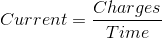If the number of charges is expressed in coulombs (C), and time in seconds (s), the current flow will have units of C/s. Like many quantities in physics, a derived unit was created to express current flow because this concept is used so often. The derived unit used to express current flow is the ampere (A), named after André-Marie Ampère, a French scientist who explored many aspects of electromagnetism. Note that roughly 6.2 billion billion electrons are needed to make up a charge of -1 C, so a current flow of even 1 ampere requires the movement of a tremendous amount of electrons past a particular point in a circuit each second.

When using equations to analyze current flow in electric circuits, it is given the symbol I.

Electric current in a circuit can be measured using an ammeter. The circuit needs to be broken at the point the measurement is to be made, and then reconnected through the ammeter, so that it can measure the current flow at that point. For example, to measure the current flow at a point between the light bulb and battery in a circuit, the ammeter would be connected as follows:

# Potential Difference (a.k.a. Voltage)

Much like we can quantify the change in gravitational potential energy of an object, like a tennis ball as it moves from one height to another in a mechanical system, we can also quantify the change in electric potential energy of an electric charge as it moves from one point to another in an electric circuit. This change in electric potential energy per unit charge is the potential difference between those two points in the circuit. Because the phrase “potential difference” can be a little cumbersome, the term “voltage” is usually used as a synonym. Mathematically this can be expressed as:If energy has units of joules (J) and charge has units of coulombs (C), the units for potential difference are J/C. Potential difference is a commonly used quantity when analyzing electric circuits so it has been given the derived unit volts (V), named after Alessandro Volta, an Italian scientist who is credited with inventing the battery.

When using equations to analyze potential difference in electric circuits, it is given the symbol V.

Potential difference in a circuit can be measured using a voltmeter. The circuit does not need to be broken to connect a voltmeter. Instead, the voltmeter is simply connected between the two points in the circuit which the potential difference is to be measured. For example, to measure the voltage across a light bulb in a circuit, the ammeter would be connected as follows:

# Resistance

Resistance is a property of devices in an electric circuit that impede current flow. Making a rough analogy between electric current flow and fluid flowing in a pipe, you can think of electric current as being the speed with which the fluid is moving, voltage as a force (or pressure differential) pushing the fluid along, and resistance as an obstacle that the fluid must pass before continuing. In an electric circuit, resistance is the only thing stopping the flow of current (and electrons in the opposite direction) from running out of control.

When electric current flows through a circuit component with resistance, its electric potential energy decreases. The principle of conservation of energy holds as the electric potential energy is converted into thermal energy. A voltmeter measures this difference in energy between current entering and exiting the component, sometimes referred to as a “voltage drop.”

In reality, all circuit components will exhibit some form of resistance, including switches, batteries, and wires. In our course, we will consider the resistance of these devices to be zero to simplify our circuit analyses. An ammeter will also be considered to have a resistance of zero, while a voltmeter will be considered to have an infinitely high resistance.# Superconductors

A superconductor is a special type of conductor that has no resistance to the flow of electric current. Because of the loss in potential energy that results as current flows through a component with resistance, the efficiency of the circuit is reduced. The one exception to this would be if the purpose of the component was to convert electrical energy into thermal energy, e.g., the element in an electric tea kettle, but even in this case the other components of the circuit (battery or generator, wires, etc.) would waste some of the electrical energy as they converted small amounts of it into thermal energy.

Since superconductors have zero resistance, they do not convert electrical energy into thermal energy as current flows through them. This means that circuits can be built with very high efficiencies. In addition, superconducting electromagnets can generate extremely strong magnetic fields that can be used in applications such as MRI machines and particle accelerators.

While superconductors are very useful, the catch is that to achieve superconductivity, a conductor must be cooled to extremely low temperatures. The exact temperature is dependent on the material the conductor is composed of, but most conductors need temperatures near -200 ºC before it is possible to exhibit superconductivity.

# Electrical Safety

Because electric current in a circuit carries electric potential energy, the design of the circuit must ensure that the “release” or conversion of this potential energy into other forms of energy is strictly controlled to avoid malfunctions, damage to property, injuries, and even death.

The most basic precaution that must be taken when building an electric circuit is to keep the conductors isolated from each other (unless, by design, they need to be connected) and other objects. This can be done through maintaining an air gap between them, like wires at different heights on a hydro pole, or by wrapping or sheathing them in a non-conductive material, insulating them from each other.

Fuses and circuit breakers protect conductors from damage due to too much current flowing. Recall that all circuit components, including wires, have some resistance. If the current flow is allowed to get too high, the buildup of thermal energy can melt the insulation around the conductors or even result in a fire. A fuse or circuit breaker in a circuit protects the wires from this sort of damage. Turning off a circuit breaker also cuts the supply of electrical energy to a circuit, making it safe to work on.

“Arc fault” circuit breakers have an additional feature: they cut the supply of electrical energy to a circuit when there is too much current flowing (like a conventional breaker) but also when the current flow characteristics match that of an arc (or spark) somewhere in the circuit. This type of circuit breaker can detect when a conductor has a small break in it to create the arc, and shutdown the supply of electrical energy to prevent a fire.

Modern household electrical circuits include both a “hot” wire that supplies electrical energy and a “neutral” wire to provide a return path for electric current, as well as a “ground” connection. The ground wire provides an additional path for current to follow as it returns to the breaker panel at a lower potential. Ground wires are connected to boxes housing electrical devices like switches, outlets and light fixtures, as well as to the housing of appliances. The use of a ground wire provides an extra layer of protection. If there is a fault somewhere along an electrical circuit, at least the current will have an easy return path to blow a fuse or trip a circuit breaker, cutting power to the circuit. The alternative would be that the electric current seeks another path, possibly through another object (creating a risk of fire) or through a person (creating a life safety risk).

A ground fault circuit interrupter (GFCI) is another type of circuit breaker that detects small differences between the current flow through the hot and neutral wires. Regular circuit breakers are great at cutting the power if the current flow is too high for the wire, but cannot guarantee safety in all situations. For example, a typical household circuit breaker might trip if the current flow exceeds 15 A, but painful shocks can result from only 0.01 A, while death is likely to result from a shock of 0.1 A. If a current of 10 A is flowing through the hot wire, and only 9.9 A is flowing through the neutral wire, a regular circuit breaker will not break the circuit, but a GFCI will. The GFCI detects the “leak” of current and breaks the circuit to avoid injury and death.

# Ohm’s Law

Electrical resistance in many devices can be quantified using Ohm’s Law. Ohm’s law is named after Georg Ohm, a German scientist who studied electricity and in particular the property of resistance of a material. Ohm’s Law relates the current flowing through a device and the voltage drop across it to a constant that is a property of the device. Mathematically, Ohm’s Law can be stated:Where V is the voltage drop in volts (V), I is the current flow in amperes (A), and R is the resistance in ohms (Ω). A resistor is a good example of a device that obeys Ohm’s Law. A light bulb is a device that does not obey Ohm’s Law – the resistance of the filament in a light bulb increases as its temperature increases.

##Example 1

Calculate the voltage drop across a resistor with a resistance of 220 Ω and a current of 1.2 A.

Given:

R = 220 Ω

I = 1.2 A

Required:

We are being asked to find the voltage drop, V.

V = ?

Analyze:

Since we know the resistance and current flow, and are being asked to find the voltage drop, we can use Ohm’s law to solve this problem.

Solve: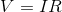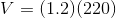Paraphrase:

The voltage drop across the resistor is 260 volts.

##Example 2

Calculate the current through a resistor with a resistance of 470 Ω that has a voltage drop of 4.5 V.

Given:

R = 470 Ω

V = 4.5 V

Required:

We are being asked to calculate the current flow through the resistor, I.

I = ?

Analyze:

Since we know the resistance and voltage drop, and are being asked to find the current flow, we can use Ohm’s Law to solve this problem. We’ll need to re-arrange the Ohm’s Law formula first: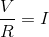Solve:Paraphrase:

The current through the resistor is 9.6 mA.# Summary:

Matter is made up of three subatomic building block particles:

Particle Mass Charge Location
Electron About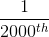that of a neutron or proton Negative Orbits the nucleus
Neutron About the same as a proton Neutral In the nucleus
Proton About the same as a neutron Positive In the nucleus

Conventional current flows from positive to negative in a circuit while electrons flow from negative to positive.

Direct current always flows in the same direction while alternating current changes direction many times per second. In both cases, the electrons always flow opposite the direction of the current.

Current flow is measured by disconnecting the circuit at the point of measurement and connecting it through an ammeter, and is recorded with the derived unit amperes (A). In an equation, current flow is represented by the symbol I. Current flow is analogous to the speed of a fluid moving through a pipe.

Potential difference or voltage is measured by connecting a voltmeter between two points in a circuit, and is recorded with the derived unit volts (V). In an equation, voltage is represented by the symbol V. Voltage is analogous to a pressure differential pushing a fluid through a pipe.

Resistance is a property of circuit components to impede current flow. Resistance has units of ohms (Ω), and is represented in an equation by the symbol R. Resistance is analogous to a barrier in a pipe that acts to impede the flow of fluid.

Many devices and procedures are necessary to ensure safety around electricity. A very small amount of current is able to cause serious injury and death.

Ohm’s Law relates the current flow through a resistor (I), the voltage drop across the resistor (V) and the resistance of the resistor (R):test text.